# 2021UKChO英国化学奥林匹克竞赛真题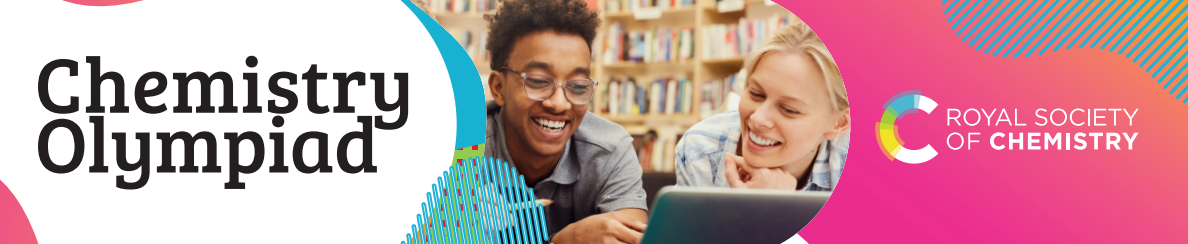UKChO报名截止时间为1月12日，有意向参赛的同学，不要错过报名时间啦！UK Chemistry Olympiad support resources: introductory questions

The following resources were developed in collaboration with Up Learn. Up Learn is an all-in-one online education platform that uses AI, applied  cognitive science and world-class educators to help A-level students  achieve A* results. To find out more, visit uplearn.co.uk.

1. This question is about air

Hundreds of years ago, scientists believed that air was a pure substance which, alongside earth, wind and fire, made up all matter on Earth.

Nowadays, we know that air is a mixture of gases.

1 mole of air contains:

0.78 moles of nitrogen

0.21 moles of oxygen

0.01 moles of argon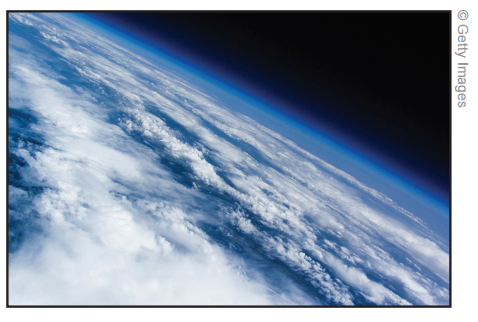(a)

(i) Using the periodic table attached, calculate the relative molecular mass of N2 and O2 , and the relative atomic mass of Ar.

(ii) Hence, calculate the mass of 1 mole of air. Give your answer to three significant figures.

(If you do not get an answer to this question, use 30.0 g in further calculations.)

The first layer of the Earth’s atmosphere, called the troposphere, ends approximately 10 km above the ground.

At standard room temperature and pressure, 1 mole of gas occupies a volume of 24 dm3.

(b)

(i) Assuming that the earth is a perfect sphere with a radius of 6371 km, estimate the volume of gas in the troposphere. Give your answer in dm3.

(ii) Hence, assuming that the gas in the troposphere is at standard room temperature and pressure, estimate the mass of the air in the troposphere.

When magnesium burns in air it reacts with oxygen to form magnesium oxide.

(c)

(i) Write a balanced equation for the reaction of magnesium with oxygen.

(ii) What is the minimum volume of air required to react with 10 g of magnesium? (Assume the reaction takes place at standard temperature and pressure.)

At higher temperatures, magnesium will also react with nitrogen gas in air to make magnesium nitride.

At 1000o C, 100 g of magnesium reacts completely in air forming a mixture of magnesium nitride and magnesium oxide. The mass of the mixture is 160 g.

Of the 100 g of magnesium that reacts, x g react to form magnesium oxide.

(d)

(i) Write an equation for the reaction of magnesium with nitrogen.

(ii) In terms of x, what mass of magnesium reacts to form magnesium nitride?

(iii) In terms of x, what mass of magnesium oxide and magnesium nitride form?

(iv) Hence, calculate the mass of magnesium that reacted with nitrogen.

1. This question is about bromine and its isotopes

At room temperature, bromine exists as a red-brown liquid with an unpleasant smell. Bromine’s smell is so unpleasant that the name bromine derives from the Ancient Greek word βρώμα which means stink.

On Earth, there are two common isotopes of bromine:

50% of bromine atoms are bromine-79

50% of bromine atoms are bromine-81

This means that molecules containing bromine can have different masses. For example, diatomic bromine, Br2 , has three possible masses:

Br2 made of two bromine-79 atoms has a mass of 158

Br2 made of one bromine-79 atom and one bromine-81 atom has a mass of 160

Br2 made of two bromine-81 atoms has a mass of 162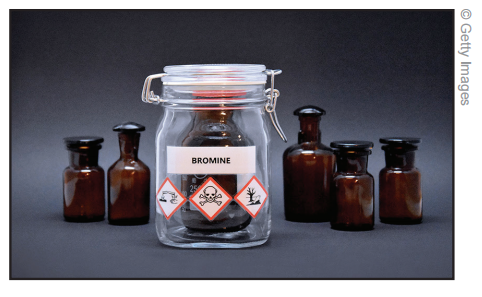(a)

(i) Calculate the probability that a Br2 molecule has a mass of 158

(ii) Calculate the probability that a Br2 molecule has a mass of 162

(iii) Hence, calculate the probability that a Br2 molecule has a mass of 160

The imaginary planet Alpha-Zar contains the same isotopes of bromine as Earth, but in different abundances. On Alpha-Zar, 36.0% of Br2 molecules have a mass of 158.

(b) What are the abundances of bromine-79 and bromine-81 atoms on Alpha-Zar?

Back on Earth, bromine atoms and boron atoms can combine to form molecules of boron tribromide, BBr3.

On Earth, there are two common isotopes of boron:

20% of boron atoms are boron-10

80% of boron atoms are boron-11

(c)

(i) List the possible masses of BBr3

(ii) What is the probability (on Earth) that a BBr3 molecule contains no 79Br atoms?

(iii) What is the probability (on Earth) that a BBr3 molecule contains exactly one 81Br atom?

(iv) What is the probability (on Earth) that a BBr3 molecule has a mass of 250?

Bromine can be used as part of a series of reactions which transform propanone (a ketone that’s used in nail polish remover) into ethyl ethanoate (an ester that’s used to decaffeinate tea and coffee).

The process is shown below: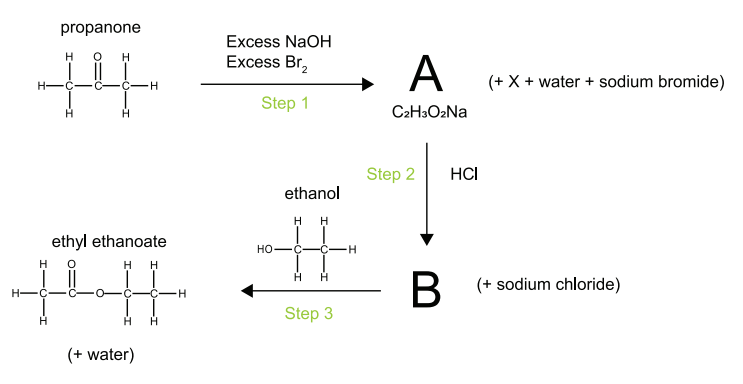(d)

(i) What is the molecular formula of propanone?

(ii) Suggest structures for A (whose formula is C2H3O2 Na) and B.

In step one, for every 1 mole of propanone that reacts, 1 mole of A and 1 mole of molecule X form.

Molecule X contains only carbon hydrogen and bromine atoms. Assuming hydrogen and carbon have only one isotope, molecule X has four possible masses.

(e) What is the molecular formula of X?

Further challenge

Challenge your understanding of atmospheric chemistry further with UK Chemistry Olympiad 2019 round 1 question 1 and UK Chemistry Olympiad 2017 round 1 question 2.

Challenge your understanding of isotopes further with UK Chemistry Olympiad 2018 round 1 question 3.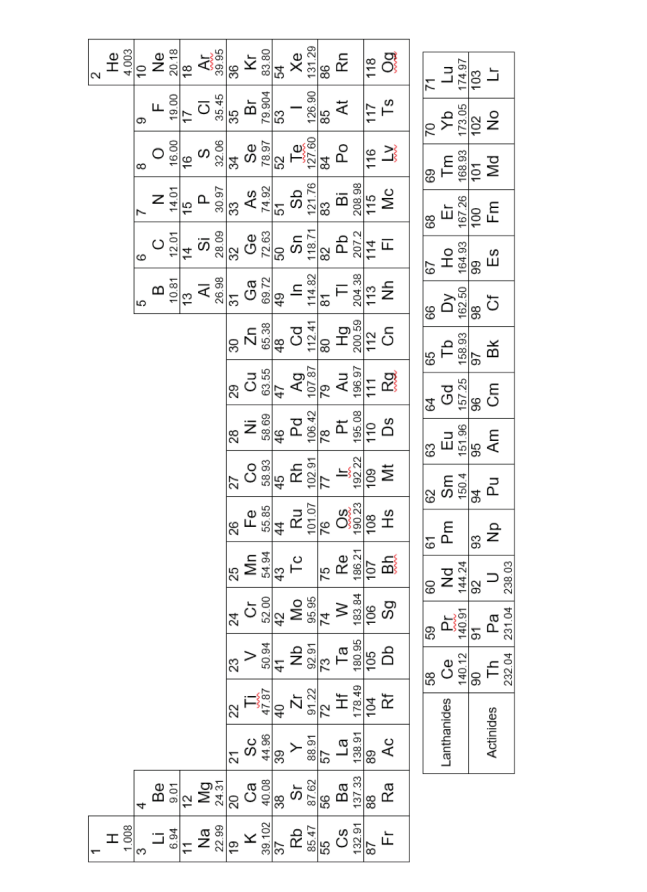### 翰林化学竞赛课程体系流程图